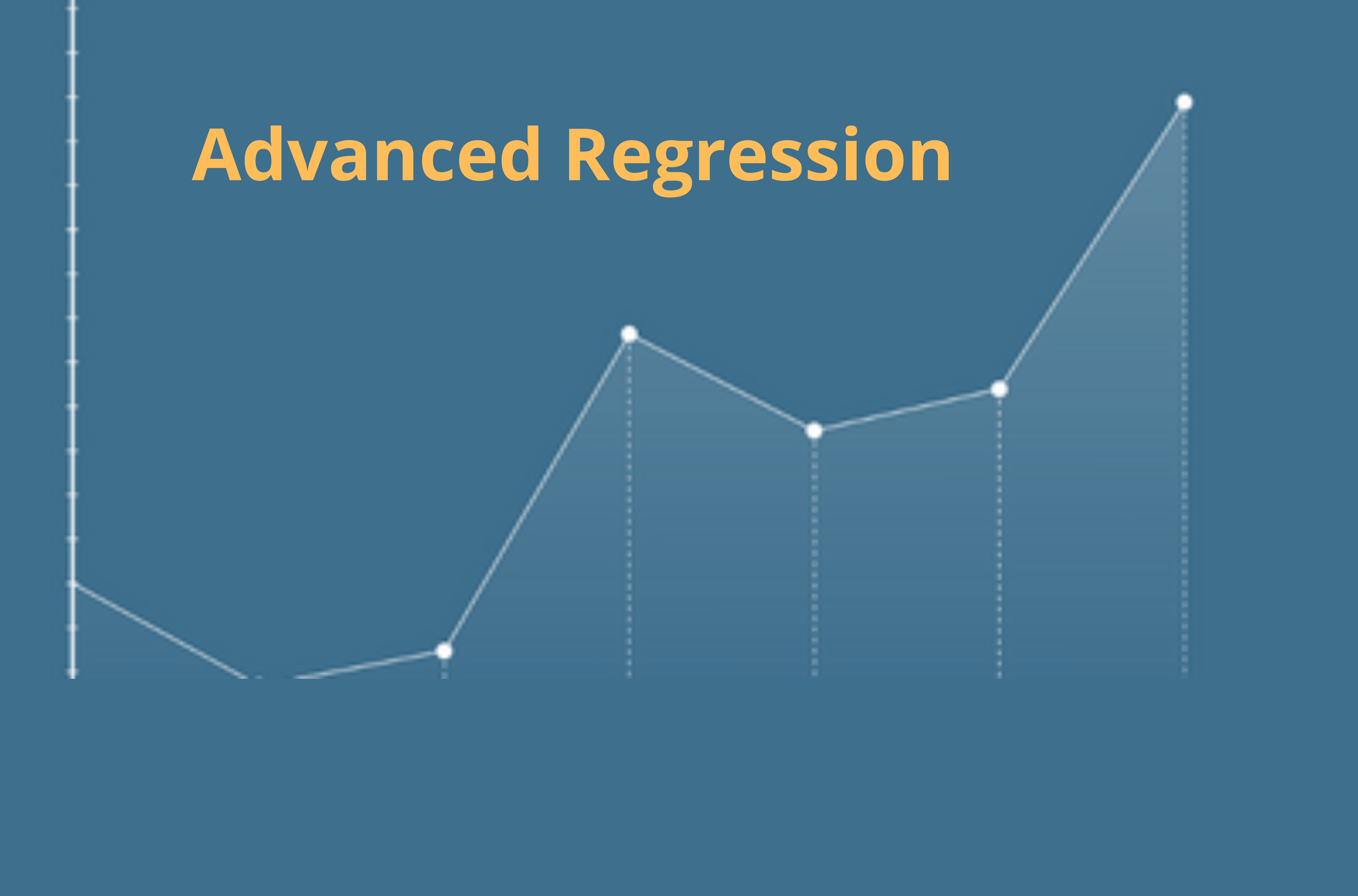• by Team Handson
• July 18, 2022

NORMAL EQUATION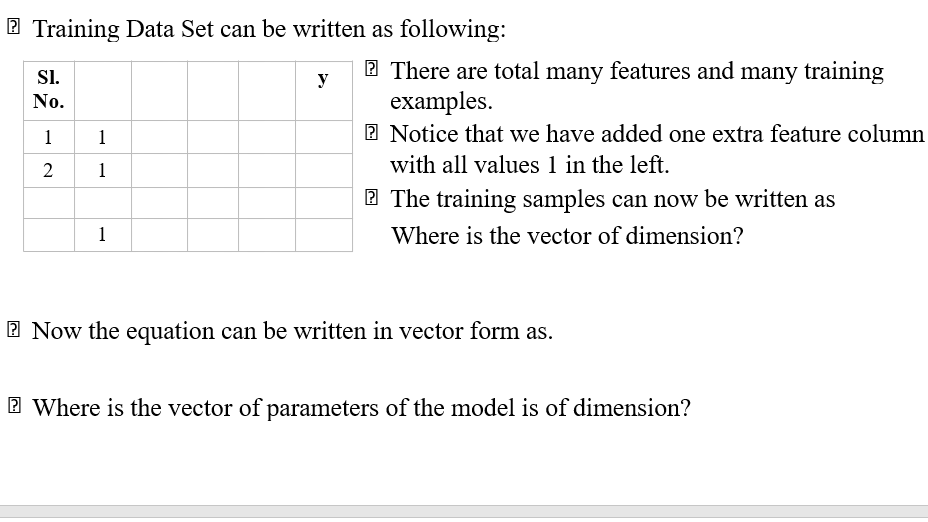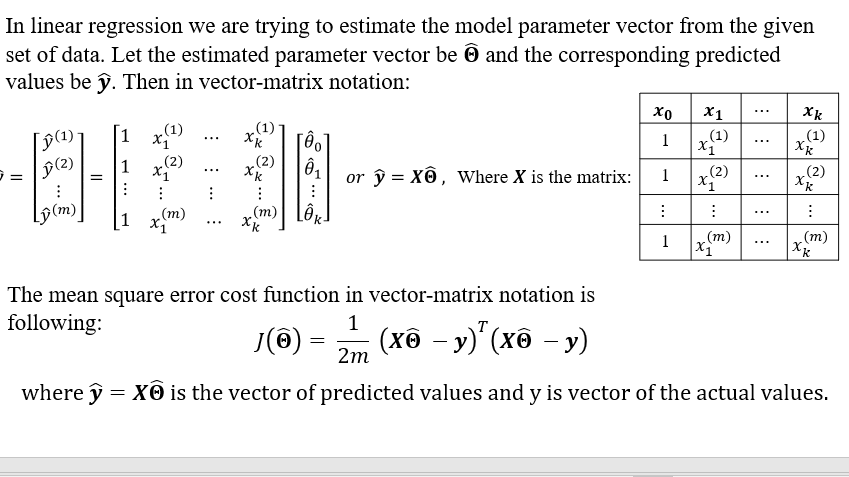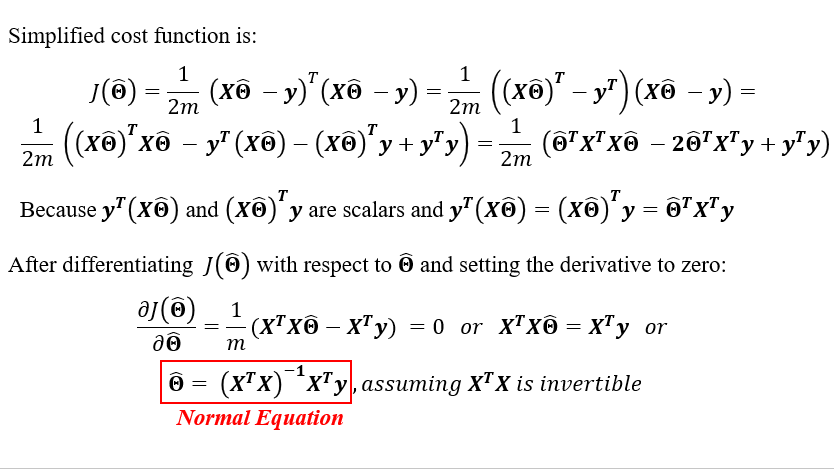• Gradient Descent or Normal Equation which one is preferable?

Though normal equation directly gives solution without iteration like GD, it has many drawbacks. Like, for large datasets computing (X^T X)^(-1) is a costly operation. Moreover, if X^T X is non-invertible we can’t use normal equation directly as above.

The workaround in the case when X^T X is non-invertible is to use pseudo-inverse. Hence, gradient descent is more popular and good choice for solving linear regression problem.Intro to Data Science: Your Step-by-Step Guide To Starting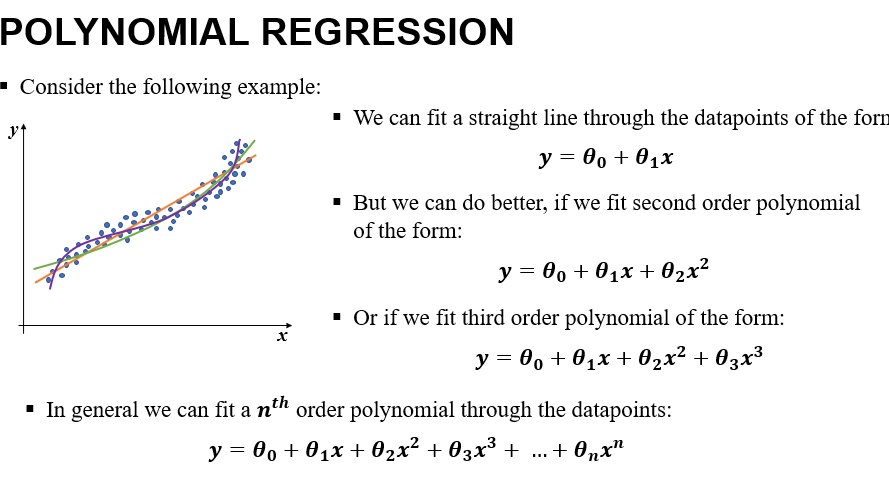• Smaller the value of n, the complexity of the model is less but the model may not fit the dataset appropriately. So we have to choose n accordingly such that we get reasonably good fit with less complexity.

We can convert the polynomial regression problem into multiple linear regression problem just by assigning:

x1=x, x2=x2, x3=x3, …, xn=xn  and then constructing multiple linear regression model   y=θ_0+ 2_(i=1)^nã€–θ_i x_i ã€—

• For more than one predictor variables the polynomial regression becomes more complicated. For two predictor variables x_1and x_2the generalized form of second order polynomial is: y=θ_0+θ_1 x_1+θ_2 x_2+θ_3 x_1 x_2+θ_4 x_1^2+θ_5 x_2^2

COEFFICIENT OF DETERMINATION

To determine the “goodness” of the fit in a linear regression model we use a quantitative measure. That is “Coefficient of Determination” (R^2). It is defined as follows.

Let there are m number of data points. y=[y_1, y_2, y_3, …, y_m ]^T is the vector of the actual values of target variable and y Ì‚=[y Ì‚_1, y Ì‚_2,y Ì‚_3, …, y Ì‚_m ]^T is the vector of predicted values of the target variable.

Let, y Ì… is the mean of the target variable. Then the Total Sum of Squares (TSS) is defined as follows:

TSS= ∑_(i=1)^mâ–’(y_i  – y Ì… )^2

TSS is proportional to the variance of the target variable.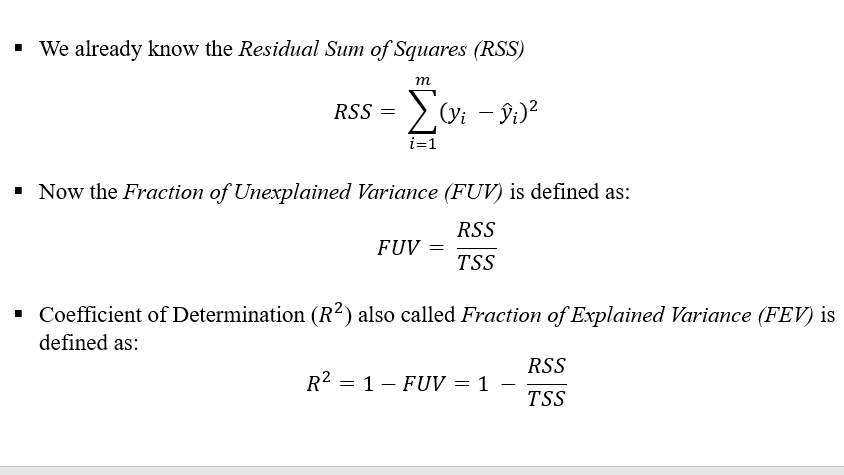Properties of Coefficient of Determination:

• Coefficient of Determination (R^2) lies between 0 to 1
• Closer the value of R^2 to 1, Regression model fits better to our datasets and can better explain the observed variability of the target variable.
• Smaller value of R^2 implies that the regression model is not that good.
• It can be shown that for bivariate dataset

R^2=Square of the correlation coefficient between the predictor and target variable.Cluster Analysis and Unsupervised Machine Learning in Python